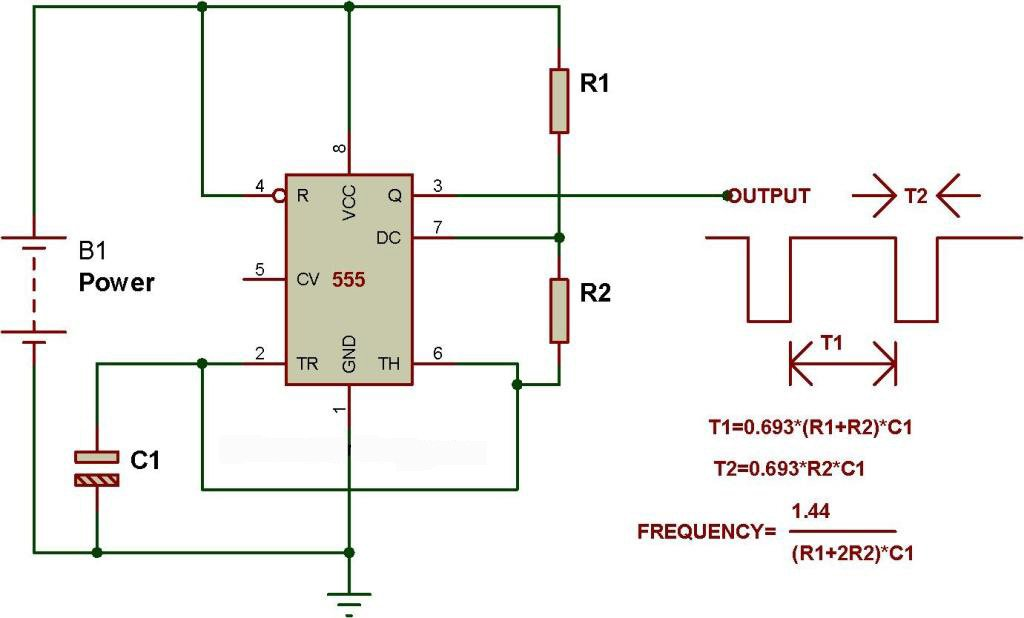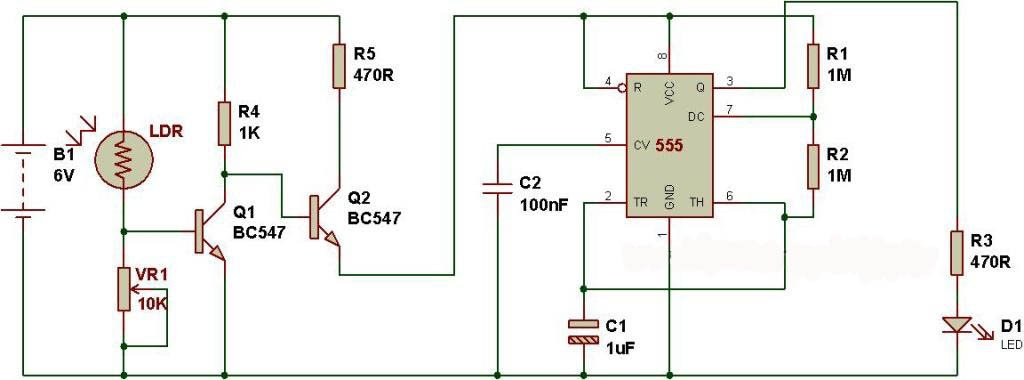This circuit has been configured in Astable mode. The output pulses are determined by the values of resistors R1, R2 and the timing capacitor C1. The formula for the frequency of pulses is: f= 1.44/[(R1+2R2)*C1].

The high and low time of each pulse can also be calculated.

High time= 0.69(R1+R2)*C1 and Low time= 0.69(R2*C1)